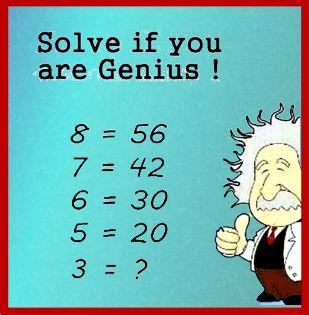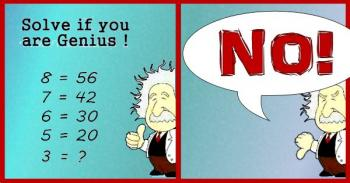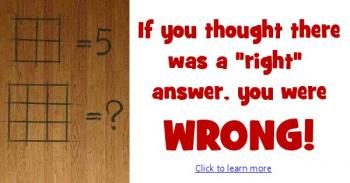Games
Problems
Go Pro!

The image below has been showing up in various incarnations on the internet. Sometimes with an image of Albert Einstein, sometimes with an image of Sheldon from "Big Bang Theory."I especially find this meme ridiculous when it's accompanied by a picture of Sheldon posing this as a problem for Penny, because anyone who knows Sheldon, and knows anything about math, know perfectly well that Sheldon would never, ever, ever pose a problem like this. Instead, he would, like good ol' Albert in the image below, give it an emphatic thumbs down.What would Sheldon say the answer is?

He would say, "Three. The answer is three. Bazinga!"

Einstein probably wouldn't say "Bazinga," but he would agree. The answer is three.

"But," someone might protest to Sheldon, "That doesn't fit the pattern!"

"Oh," Sheldon would say, "You want a pattern? Okay. The answer is the square root of seventeen."

"No," you would say, "That's wrong."

"Exactly," Sheldon would say. "You wanted me to create a pattern. The first four equations are wrong, so I made another wrong equation to complete the pattern. Bazinga again!"

Einstein would probably answer differently. He would probably say, "Just because the first four equations are wrong doesn't mean I have to be wrong, too. The answer is three."

You see, in mathematics, the equals sign has a very specific meaning; it means that the two sides of the equation are equal. So I don't care if the person who made this meme got four equations wrong, the answer is still three.

What the problem writer probably meant to do was to use function notation:

f(8) = 56
f(7) = 42
f(6) = 30
f(5) = 20
f(3) = ?

Now we at least have valid mathematical notation. But is the problem solvable? Not really, no. Because there isn't just one function which satisfies the values above, and not all of them produce the same value for f(3).

For example, the function that the image creator probably wanted you to use was: f(x) = x- x, which works for all the values given, and gives f(3) = 32 - 3 = 6. This is the answer most people are accepting as correct. However, consider the following as an alternative function:

f(x) = (x3 - 4x+ 3x)/(x - 3)

With this function, we get the correct values but we can't plug three into the function because it results in a divsion by zero!

So, even if we fix the notation, the real geniuses will tell you that it can't be solved.

Bazinga.

• Phil wanted to know if I could find a function that matched all the data points in the pattern. but produced a different value for f(3). (Apparently he thought it was cheating to create a function that has no value for f(3)!). Anyway, the answer is, yes, I can do it, and here's the explanation of how: Creating Einstein's Meme Function.
• Toward the end here I'm being a bit tongue in cheek, pointing out that there isn't just one solution. For most of us, though, if we can find a pattern that matches four data points, we feel like we've come up with a satisfactory "solution."  It's a whole different matter if you're given only one data point and asked to find a pattern, which is what happens in the puzzle linked below. Be sure to read about the "squares" problem for more about that!

Click the image below for another question and answer!Like us on Facebook to get updates about new resources